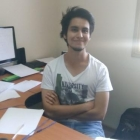﻿﻿ CI²MA - Publicaciones | Tesis de Pregrado

# Tesis de Pregrado de Sebastián MoragaCarreraIngeniería Civil Matemática, Universidad de Concepción
Año de Ingreso2013
Año de Egreso2019
Título de la TesisAnálisis basado en espacios de Banach de un nuevo método de elementos finitos completamente mixto para el problema de Boussinesq

#### Resumen de la Tesis:

In this work we propose and analyze, utilizing mainly tools and abstract results from Banach spaces rather than from Hilbert ones, a new fully-mixed finite element method for the stationary Boussinesq problem with temperature-dependent viscosity. More precisely, following an idea that has already been applied to the Navier-Stokes equations and to the fluid part only of our model of interest, we first incorporate the velocity gradient and the associated Bernoulli stress tensor as auxiliary unknowns. Additionally, and differently from earlier works in which either the primal or the classical dual-mixed method is employed for the heat equation, we consider here an analogue of the approach for the fluid, which con- sists of introducing as further variables the gradient of temperature and a vector version of the Bernoulli tensor. The resulting mixed variational formulation, which involves the afore- mentioned four unknowns together with the original variables given by the velocity and temperature of the fluid, is then reformulated as a fixed point equation. Next, we utilize the well-known Banach and Brouwer theorems, combined with the application of the Babuška- Brezzi theory to each independent equation, to analyze the solvability of the continuous and discrete schemes. In particular, Raviart-Thomas spaces of order k ≥ n − 1 for the Bernoulli tensor and its vector version for the heat equation, and piecewise polynomials of degree ≤ k for the velocity, the temperature, and both gradients, become a feasible choice. Finally, we derive optimal a priori error estimates and provide several numerical results illustrating the performance of the fully-mixed scheme and confirming the theoretical rates of convergence.

Director(es) de Tesis Eligio Colmenares, Gabriel N. Gatica
Fecha de Aprobación Proyecto de Tesis2018, Enero 08
Fecha de Defensa de Tesis2019, Marzo 08
Seguimiento Profesional
PDF TesisDescargar Tesis en PDF(no hay publicaciones)

<< Volver a listado de Tesis de Pregrado.